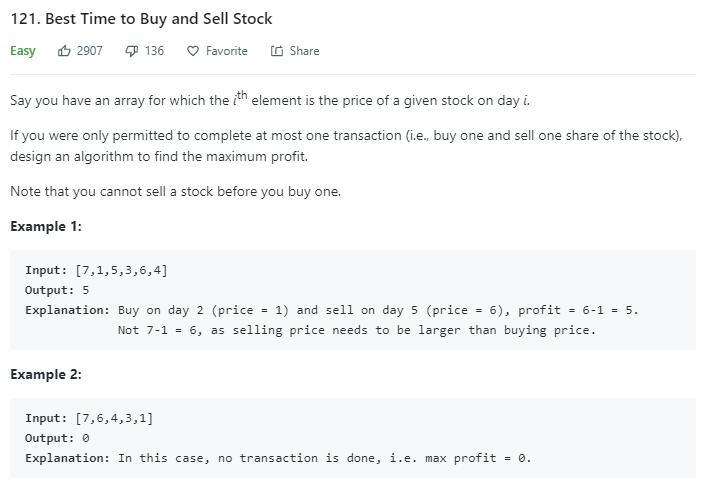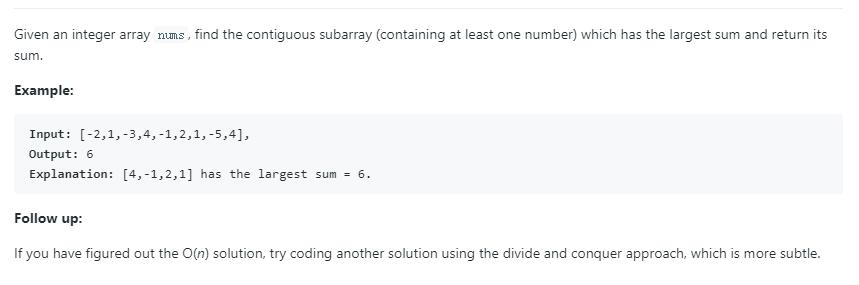# 题目描述（简单难度）# 解法一 暴力破解

public int maxProfit(int[] prices) {
int maxProfit = 0;
for (int i = 0; i < prices.length; i++) {
for (int j = i + 1; j < prices.length; j++) {
maxProfit = Math.max(maxProfit, prices[j] - prices[i]);
}
}
return maxProfit;
}


# 解法二 双指针

用两个指针， buy 表示第几天买入，sell 表示第几天卖出

3 6 7 2 9
^
b
^
s

sell 后移表示这天卖出，计算收益是 6 - 3 = 3
3 6 7 2 9
^ ^
b s

sell 后移表示这天卖出，计算收益是 7 - 3 = 4
3 6 7 2 9
^   ^
b   s

sell 后移表示这天卖出，计算收益是 2 - 3 = -1
3 6 7 2 9 12
^     ^
b     s

3 6 7 2 9 12
^
s
^
b

sell 后移表示这天卖出，计算收益是 9 - 2 = 7

3 6 7 2 9 12
^ ^
b s

sell 后移表示这天卖出，计算收益是 12 - 2 = 10
3 6 7 2 9 12
^   ^
b   s



public int maxProfit(int[] prices) {
int maxProfit = 0;
int sell = 0;
for (; sell < prices.length; sell++) {
} else {
maxProfit = Math.max(maxProfit, prices[sell] - prices[buy]);

}
}
return maxProfit;
}


# 解法三• 如果 dp [ i - 1 ] < 0，那么 dp [ i ] = nums [ i ]
• 如果 dp [ i - 1 ] >= 0，那么 dp [ i ] = dp [ i - 1 ] + nums [ i ]

public int maxSubArray(int[] nums) {
int n = nums.length;
int dp = nums;
int max = nums;
for (int i = 1; i < n; i++) {
dp= Math.max(dp + nums[i],nums[i]);
max = Math.max(max, dp);
}
return max;
}


public int maxProfit(int[] prices) {
int n = prices.length;
int dp = 0;
int max = 0;
for (int i = 1; i < n; i++) {
int num = prices[i] - prices[i - 1];
dp = Math.max(dp + num, num);
max = Math.max(max, dp);
}
return max;
}